Home | | Transforms and Partial Differential Equations | Important Questions and Answers: Partial Differential Equations

# Important Questions and Answers: Partial Differential Equations

Mathematics (maths) - Partial Differential Equations

PARTIAL DIFFERENTIAL EQUATIONS

1. Explain how PDE are formed?

PDE can be obtained

(i)          By eliminating the arbitrary constants that occur in the functional relation between the dependent and independent variables.

(ii)       By eliminating arbitrary functions from a given relation between the dependent and independent variables.

2.From the PDE by eliminating the arbitrary constants a & b from z =ax  +by .

Given  z =ax +by

Diff. p.w.r. to x we get,3. From the PDE by eliminating the arbitrary constants a & b from5. Obtain PDE from     z =f (sin x + cos y) .

Given   z =f (sin x  +cos y) â€¦(1)Integrating w.r to x on both sides

z = -cos x  c+

But z is a function of x and  y

\  z =-cos x   f+(y)

Hence c =f ( y) .

7. Mention three types of solution of a p.d.e (or) Define general and complete integrals of a

p.d.e.

(i)    A solution which contains as many arbitrary constants as there are independent variables is called a complete integral (or) complete solution.

(ii) A solution obtained by giving particular values to the arbitrary constants in a complete integral is called a particular integral (or) particular solution.

(iii)A solution of a p.d.e which contains the maximum possible number of arbitrary functions is called a general integral (or) general solution.This is of the form F(p,q) = 0.

Hence the complete integral is z =ax  +by  cz.There is no singular integral.

Taking  c =f (a)when f is arbitrary.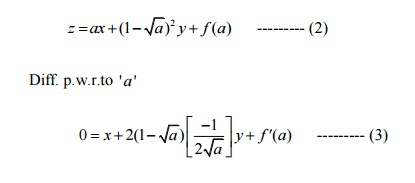Eliminating ' a '  between (2) & (3) we get the general solution.

9. Find the complete integral ofThis equation is of the form   z =px  +qy   f+(p, q) .

By   Clairautâ€™s type,put p=a , q =b.

Therefore the complete integral is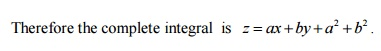10. Find the complete integral of     q =2 px .

Given          q =2 px .

This equation of the form        f (x, p, q) =0 .11. Find the complete integral of  pq =xy .

Given          pq =xy .It is of the form  f (x, p ) =f(y , q) .Hence dz =pdx  +qdy .The given differential equation can be written as,Where a & b are arbitrary constant.

To Find The Singular integral:

Diff (1)  p.w.r.to a,Which is the singular solution.

To Get the general integral:

Put b =f(a) in (1) , we getEliminate a between (5) abd (6) to get the general solution.

2.Solve y2p-xyq=x(z-2y)

Soln:

Given y2p-xyq=x(z-2y)

This equation of the form Pp+Qq=R

Here, P=y2 ,Q=-xy ,  R= x(z-2y)Hence the general solution is f(x2+y2 , yz-y2)=0.

3.Solve:(3z-4y)p+(4x-2z)q=2y-3x

Soln:

Given: (3z-4y)p+(4x-2z)q=2y-3x

This equation of the form Pp+q=R

Here, P= (3z-4y)   ,Q=(4x-2z) ,  R= 2y-3x

Again use Lagrangian multipliers 2,3,4,2x+3y+4z=b.

Hence the general solution is,

F(x2+y2+z2  ,  2x+3y+4z)=0.

4.Find the general solution of x(y2-z2)p+y(z2-x2)q=z(x2-y2)

Soln;

Given; x(y2-z2)p+y(z2-x2)q=z(x2-y2)

This equation of the form Pp+q=R

Here, P= x(y2-z2) ,Q= y(z2-x2) , R= z(x2-y2)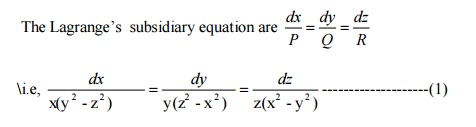Use Lagrangian multipliers x,y,z,

We get the ratio in (1)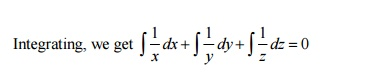logx +logy+logz=log b

Hence the general solution is,

F(x2+y2+z2  , logx +logy+logz)=0.The auxiliary equation is m3-2m2=0

Replace   D   by   m   and   Dâ€™   by   1

m2(m-2)=0

m=0,0  and m=2=4 IP (-i(cos(x +y) +i sin (x +y))

=-4 cos (x +y)

Hence the general solution is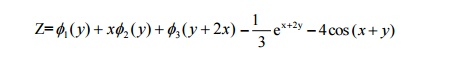Study Material, Lecturing Notes, Assignment, Reference, Wiki description explanation, brief detail
Mathematics (maths) : Partial Differential Equations : Important Questions and Answers: Partial Differential Equations |